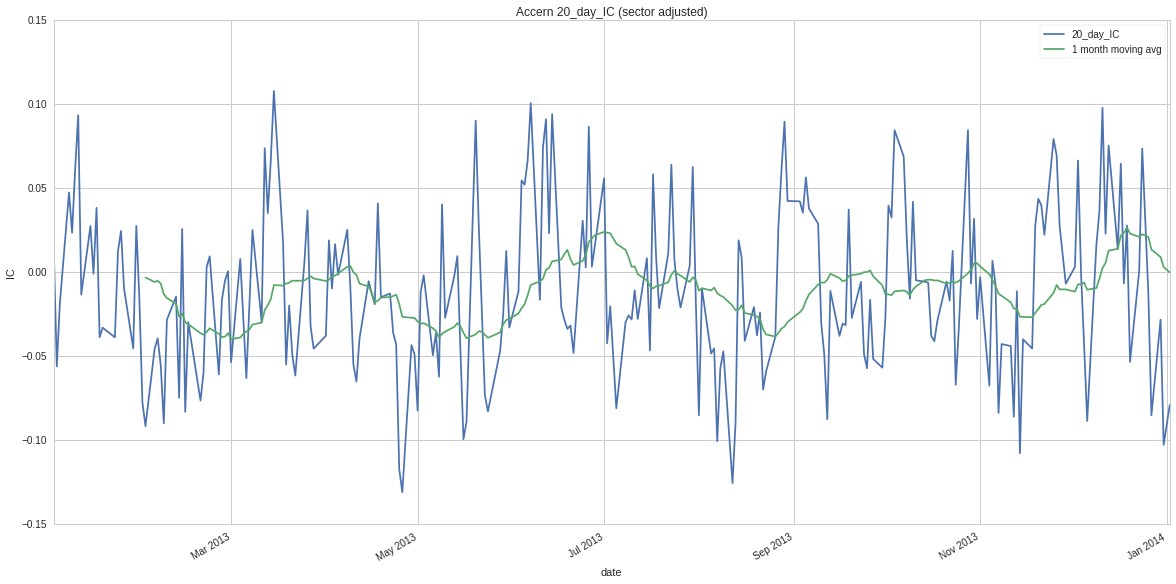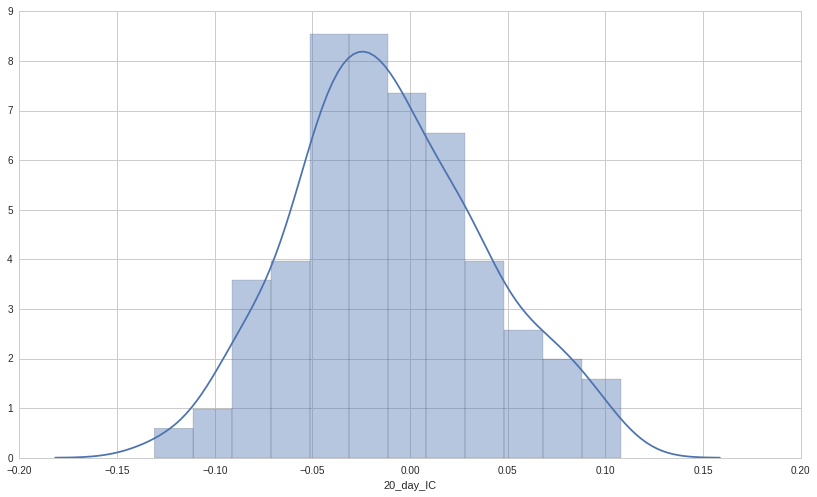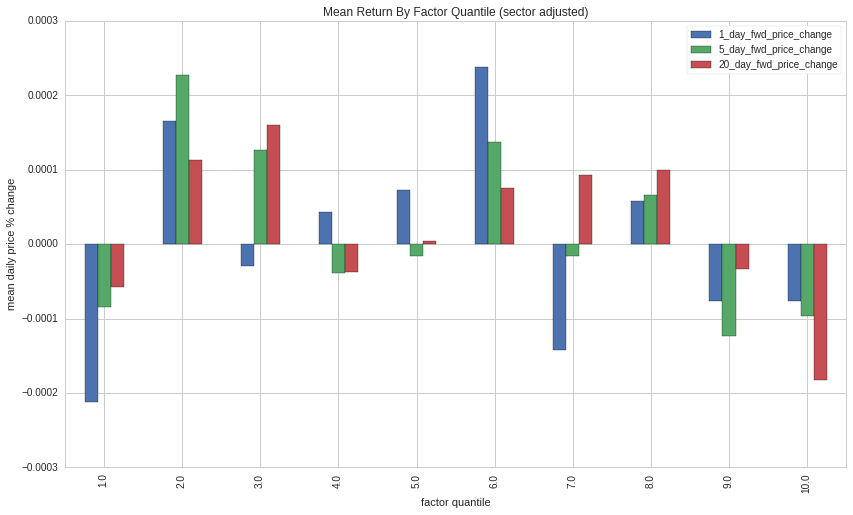Notebook

# Factor Tearsheet¶

When implementing a factor in a trading algorithm, the complexity and wide range of parameters that come with basket selection and trading logic hinder our ability to evaluate the value factor's alpha signal in isolation. Before we proceed to the implementation of an algorithm, we want to know if the factor has any predictive value.

In this analysis, we'll measure a factor's predictive value using the Spearman rank correlation between the factor value and various N day forward price movement windows over a large universe of stocks. This correlation is called the Information Coefficient (IC). This tear sheet takes a pipeline factor and attempt to answer the following questions, in order:

• What is the sector-neutral rolling mean IC for our different forward price windows?
• What are the sector-neutral factor decile mean returns for our different forward price windows?
• How much are the contents of the top and bottom quintile changing each day?
• What is the autocorrelation in sector-wise factor rankings?
• What is IC decay (difference in IC for different forward price windows) for each sector?
• What is the IC decay for each sector over time?
• What are the factor quintile returns for each sector?

For more information on Spearman Rank correlation, check out this notebook from the Quantopian lecture series.

In the plots that are not disagregated by sector, sector adjustment has been applied to forward price movements. You can think of this sector adjustment as incorperating the assumption of a sector-netural portfolio constraint. If we are equally weighted in each sector, we'd want our factor to help us compare stocks within their own sectors. For example, if AAPL 5-day forward return is 0.1% and the mean 5-day forward return for the Technology stocks in our universe was 0.5% in the same period, the sector adjusted 5 day return for AAPL in this period is -0.4%.

The autocorrelation and decile turnover figures are meant to be used as a measure of factor horizon. It is worth noting that these stats are potentially misleading, as our top X liquidity constraint makes our universe dynamic. This dynamic universe likely contributes to a higher quantile turnover and lower rank autocorrelation than we would see in a static universe.

In :
from __future__ import division
from quantopian.pipeline import Pipeline
from quantopian.pipeline import CustomFactor
from quantopian.research import run_pipeline
from quantopian.pipeline.data import morningstar
from quantopian.pipeline.data.builtin import USEquityPricing
from quantopian.pipeline.factors import Latest, SimpleMovingAverage as SMA

from quantopian.pipeline.data.psychsignal import stocktwits_free as stocktwits
from quantopian.pipeline.data.sentdex import sentiment_free as sentdex
from quantopian.pipeline.data.accern import alphaone_free as accern

import numpy as np
import pandas as pd
import scipy as sp
import pyfolio as pf
import matplotlib.pyplot as plt
import seaborn as sns

SECTOR_NAMES = {
101: 'Basic Materials',
102: 'Consumer Cyclical',
103: 'Financial Services',
104: 'Real Estate',
205: 'Consumer Defensive',
206: 'Healthcare',
207: 'Utilities',
308: 'Communication Services',
309: 'Energy',
310: 'Industrials',
311: 'Technology' ,
}

In :
class Liquidity(CustomFactor):
inputs = [USEquityPricing.volume, USEquityPricing.close]
window_length = 5

def compute(self, today, assets, out, volume, close):
out[:] = (volume * close).mean(axis=0)

class Sector(CustomFactor):
inputs = [morningstar.asset_classification.morningstar_sector_code]
window_length = 1
def compute(self, today, assets, out, msc):
out[:] = msc[-1]

def construct_factor_history(factor_cls, start_date='2015-10-1', end_date='2016-2-1',
factor_name='factor',
top_liquid=1000, universe_constraints=None, sector_names=None):
"""
Creates a DataFrame containing daily factor values and sector codes for a liquidity
constrained universe. The returned DataFrame is can be used in the factor tear sheet.

Parameters
----------
factor_cls : quantopian.pipeline.CustomFactor
Factor class to be computed.
start_date : string or pd.datetime
Starting date for factor computation.
end_date : string or pd.datetime
End date for factor computation.
factor_name : string, optional
Column name for factor column in returned DataFrame.
top_liquid : int, optional
Limit universe to the top N most liquid names each trading day.
Based on trailing 5 days traded dollar volume.
universe_constraints : num_expr, optional
Pipeline universe constraint.

Returns
-------
daily_factor : pd.DataFrame
DataFrame with integer index and date, equity, factor, and sector
code columns.

"""

factor = factor_cls()
sector = Sector()
liquidity = Liquidity()

liquidity_rank = liquidity.rank(ascending=False)
ok_universe = (top_liquid > liquidity_rank)  & factor.eq(factor) & sector.eq(sector)

if universe_constraints is not None:
ok_universe = ok_universe & universe_constraints

pipe = Pipeline()
pipe.set_screen(ok_universe)

daily_factor = run_pipeline(pipe, start_date=start_date, end_date=end_date)
daily_factor = daily_factor.reset_index().rename(
columns={'level_0': 'date', 'level_1':'equity'})

daily_factor = daily_factor[daily_factor.sector_code != -1]
if sector_names is not None:
daily_factor.sector_code = daily_factor.sector_code.apply(
lambda x: sector_names[x])

return daily_factor

def add_forward_price_movement(daily_factor, days=[1, 5, 20], prices=None):
"""
Adds N day forward price movements (as percent change) to a factor value
DataFrame.

Parameters
----------
daily_factor : pd.DataFrame
DataFrame with, at minimum, date, equity, factor, columns. Index can be integer or
date/equity multiIndex.
See construct_factor_history for more detail.
days : list
Number of days forward to project price movement. One column will be added for each value.
prices : pd.DataFrame, optional
Pricing data to use in forward price calculation. Equities as columns, dates as index.
If no value is passed, get pricing will be called.

Returns
-------
factor_and_fp : pd.DataFrame
DataFrame with integer index and date, equity, factor, sector
code columns with and an arbitary number of N day forward percentage
price movement columns.

"""
factor_and_fp = daily_factor.copy()
if not isinstance(factor_and_fp.index, pd.core.index.MultiIndex):
factor_and_fp = factor_and_fp.set_index(['date', 'equity'])

if prices is None:
start_date = factor_and_fp.index.levels.values.min()
end_date = factor_and_fp.index.levels.values.max()

equities = factor_and_fp.index.levels.unique()

time_buffer = pd.Timedelta(days=max(days)+5)
prices = get_pricing(equities,
start_date=start_date,
end_date=end_date+time_buffer,
fields='open_price')

col_n = '%s_day_fwd_price_change'
for i in days:
delta = prices.pct_change(i).shift(-i)
factor_and_fp[col_n%i] = delta.stack()

factor_and_fp = factor_and_fp.reset_index()

return factor_and_fp

"""
Convert forward price movements to price movements relative to mean sector price movements.
This normalization incorperates the assumption of a sector neutral portfolio constraint
and thus allows allows the factor to be evaluated across sectors.

For example, if AAPL 5 day return is 0.1% and mean 5 day return for the Technology stocks
in our universe was 0.5% in the same period, the sector adjusted 5 day return for AAPL
in this period is -0.4%.

Parameters
----------
factor_and_fp : pd.DataFrame
DataFrame with date, equity, factor, and forward price movement columns. Index should be integer.

Returns
-------
DataFrame with integer index and date, equity, factor, sector
code columns with and an arbitary number of N day forward percentage
price movement columns, each normalized by sector.

"""
pc_cols = [col for col in factor_and_fp.columns.values if 'fwd_price_change' in col]

lambda x: x - x.mean())

def factor_spearman_rank_IC(factor_and_fp, time_rule=None, by_sector=True, factor_name='factor'):
"""
Computes sector neutral Spearman Rank Correlation based Information Coefficient between
factor values and N day forward price movements.

Parameters
----------
factor_and_fp : pd.DataFrame
DataFrame with date, equity, factor, and forward price movement columns. Index should be integer.
time_rule : string, optional
Time span to use in Pandas DateTimeIndex grouping reduction.
See http://pandas.pydata.org/pandas-docs/stable/timeseries.html for available options.
by_sector : boolean
If True, compute ic separately for each sector
factor_name : string
Name of factor column on which to compute IC.

Returns
-------
ic : pd.DataFrame
Spearman Rank correlation between factor and provided forward price movement columns.
MultiIndex of date, sector.
err : pd.DataFrame
Standard error of computed IC. MultiIndex of date, sector.
MultiIndex of date, sector.

"""
def src_ic(x):
cn = "%s_day_IC"
ic = pd.Series()
for days, col in zip(fwd_days, pc_cols):
ic[cn%days] = sp.stats.spearmanr(x[factor_name], x[col])
ic['obs_count'] = len(x)

return ic

def src_std_error(rho, n):
return np.sqrt((1-rho**2)/(n-2))

fwd_days, pc_cols = get_price_move_cols(factor_and_fp)

grpr = ['date', 'sector_code'] if by_sector else ['date']
ic = factor_and_fp.groupby(grpr).apply(src_ic)

obs_count = ic.pop('obs_count')
err = ic.apply(lambda x: src_std_error(x, obs_count))

if time_rule is not None:
ic = ic.reset_index().set_index('date')
err = err.reset_index().set_index('date')

grpr = [pd.TimeGrouper(time_rule),'sector_code'] if by_sector else [pd.TimeGrouper(time_rule)]
ic = ic.groupby(grpr).mean()
err = err.groupby(grpr).agg(
lambda x: np.sqrt((np.sum(np.power(x, 2))/len(x))))

else:
if by_sector:
ic = ic.reset_index().groupby(['sector_code']).mean()
err = err.reset_index().groupby(['sector_code']).agg(
lambda x: np.sqrt((np.sum(np.power(x, 2))/len(x))))

return ic, err

def quantile_bucket_factor(factor_and_fp, by_sector=True, quantiles=5, factor_name='factor'):
"""
Computes daily factor quantiles.

Parameters
----------
factor_and_fp : pd.DataFrame
DataFrame with date, equity, factor, and forward price movement columns.
Index should be integer. See add_forward_price_movement for more detail.
by_sector : boolean
If True, compute quantile buckets separately for each sector.
quantiles : integer
Number of quantiles buckets to use in factor bucketing.
factor_name : string
Name of factor column on which to compute quantiles.

Returns
-------
factor_and_fp_ : pd.DataFrame
Factor and forward price movements with additional factor quantile column.
"""

g_by = ['date', 'sector_code'] if by_sector else ['date']
factor_and_fp_ = factor_and_fp.copy()

factor_and_fp_['factor_percentile'] = factor_and_fp_.groupby(
g_by)[factor_name].rank(pct=True)

q_int_width = 1. / quantiles
factor_and_fp_['factor_bucket'] = factor_and_fp_.factor_percentile.apply(
lambda x: ((x - .000000001) // q_int_width) + 1)

return factor_and_fp_

def quantile_bucket_mean_daily_return(quantile_factor, by_sector=False):
"""
Computes mean daily returns for factor quantiles across provided forward
price movement columns.

Parameters
----------
quantile_factor : pd.DataFrame
DataFrame with date, equity, factor, factor quantile, and forward price movement columns.
Index should be integer. See quantile_bucket_factor for more detail.
by_sector : boolean
If True, compute quintile bucket returns separately for each sector
quantiles : integer
Number of quantiles buckets to use in factor bucketing.

Returns
-------
mean_returns_by_quantile : pd.DataFrame
Sector-wise mean daily returns by specified factor quantile.
"""
fwd_days, pc_cols = get_price_move_cols(quantile_factor)

def daily_mean_ret(x):
mean_ret = pd.Series()
for days, col in zip(fwd_days, pc_cols):
mean_ret[col] = x[col].mean() / days

return mean_ret

g_by = ['sector_code', 'factor_bucket'] if by_sector else ['factor_bucket']
mean_ret_by_quantile = quantile_factor.groupby(
g_by)[pc_cols].apply(daily_mean_ret)

return mean_ret_by_quantile

def quantile_turnover(quantile_factor, quantile):
"""
Computes the proportion of names in a factor quantile that were
not in that quantile in the previous period.

Parameters
----------
quantile_factor : pd.DataFrame
DataFrame with date, equity, factor, factor quantile, and forward price movement columns.
Index should be integer. See quantile_bucket_factor for more detail.
quantile : integer
Quantile on which to perform turnover analysis.

Returns
-------
quant_turnover : pd.Series
Period by period turnover for that quantile.
"""

quant_names = quantile_factor[quantile_factor.factor_bucket == quantile]

quant_name_sets = quant_names.groupby(['date']).equity.apply(set)
new_names = (quant_name_sets - quant_name_sets.shift(1)).dropna()
quant_turnover = new_names.apply(lambda x: len(x)) / quant_name_sets.apply(lambda x: len(x))

return quant_turnover

def factor_rank_autocorrelation(daily_factor, time_rule='W', factor_name='factor'):
"""
Computes autocorrelation of mean factor ranks in specified timespans.
We must compare week to week factor ranks rather than factor values to account for
systematic shifts in the factor values of all names or names within a sector.
This metric is useful for measuring the turnover of a factor. If the value of a factor
for each name changes randomly from week to week, we'd expect a weekly autocorrelation of 0.

Parameters
----------
daily_factor : pd.DataFrame
DataFrame with integer index and date, equity, factor, and sector
code columns.
time_rule : string, optional
Time span to use in factor grouping mean reduction.
See http://pandas.pydata.org/pandas-docs/stable/timeseries.html for available options.
factor_name : string
Name of factor column on which to compute IC.

Returns
-------
autocorr : pd.Series
Rolling 1 period (defined by time_rule) autocorrelation of factor values.

"""
daily_ranks = daily_factor.copy()
daily_ranks[factor_name] = daily_factor.groupby(['date', 'sector_code'])[factor_name].apply(
lambda x: x.rank(ascending=True))

equity_factor = daily_ranks.pivot(index='date', columns='equity', values=factor_name)
if time_rule is not None:
equity_factor = equity_factor.resample(time_rule, how='mean')

autocorr = equity_factor.corrwith(equity_factor.shift(1), axis=1)

return autocorr

def get_price_move_cols(x):
pc_cols = [col for col in x.columns.values if 'fwd_price_change' in col]
fwd_days = map(lambda x: int(x.split('_')), pc_cols)

return fwd_days, pc_cols

def get_ic_cols(x):
return [col for col in x.columns.values if 'day_IC' in col]

In :
def plot_daily_ic(factor_and_fp, factor_name='factor'):
"""
Plots Spearman Rank Information Coefficient and IC moving average for a given factor.
Sector neturalization of forward price movements with sector_adjust_forward_price_moves is
recommended.

Parameters
----------
factor_and_fp : pd.DataFrame
DataFrame with date, equity, factor, and forward price movement columns.
factor_name : string
Name of factor column on which to compute IC.
"""

daily_ic, _ = factor_spearman_rank_IC(factor_and_fp, by_sector=False,
factor_name=factor_name)
ic_cols = get_ic_cols(daily_ic)
for col in ic_cols:
mean_ic = daily_ic[col].mean()
std_ic = daily_ic[col].std()
fp_ic = pd.DataFrame(daily_ic[col])
fp_ic['1 month moving avg'] = pd.rolling_mean(fp_ic[col], 22)
fp_ic.plot(title= "{} {} (sector adjusted)".format(factor_name, col), figsize=(20,10))
print('{} mean: {}'.format(col, mean_ic))
print('{} stdev: {}'.format(col, std_ic))
print('{} mean/stdev: {}'.format(col, mean_ic/std_ic))
plt.ylabel('IC')
plt.xlabel('date')
plt.show()
sns.distplot(daily_ic[col] ,norm_hist=True)
plt.show()

def plot_ic_by_sector(factor_and_fp, factor_name='factor'):
"""
Plots Spearman Rank Information Coefficient for a given factor over provided forward price
movement windows. Separates by sector.

Parameters
----------
factor_and_fp : pd.DataFrame
DataFrame with date, equity, factor, and forward price movement columns.
factor_name : string
Name of factor column on which to compute IC.
"""
ic_sector, err_sector = factor_spearman_rank_IC(factor_and_fp, factor_name=factor_name)

ic_sector.plot(kind='bar' ) #yerr=err_sector
fig = plt.gcf()
fig.suptitle("Information Coefficient by Sector", fontsize=16, x=.5, y=.93)
plt.show()

def plot_ic_by_sector_over_time(factor_and_fp, time_rule=None, factor_name='factor'):
"""
Plots sector-wise time window mean daily Spearman Rank Information Coefficient
for a given factor over provided forward price movement windows.

Parameters
----------
factor_and_fp : pd.DataFrame
DataFrame with date, equity, factor, and forward price movement columns.
time_rule : string, optional
Time span to use in time grouping reduction.
See http://pandas.pydata.org/pandas-docs/stable/timeseries.html for available options.
factor_name : string
Name of factor column on which to compute IC.
"""
ic_time, err_time = factor_spearman_rank_IC(factor_and_fp, time_rule=time_rule,
factor_name=factor_name)
ic_time = ic_time.reset_index()
err_time = err_time.reset_index()

f, axes = plt.subplots(6,2, sharex=False, sharey=True, figsize=(20,45))
axes = axes.flatten()
i = 0
for sc, data in ic_time.groupby(['sector_code']):
e = err_time[err_time.sector_code == sc].set_index('date')
data.drop('sector_code', axis=1).set_index('date').plot(kind='bar',
title=sc,
ax=axes[i],
) # yerr=e
i+=1
fig = plt.gcf()
fig.suptitle("Monthly Information Coefficient by Sector", fontsize=16, x=.5, y=.93)
plt.show()

def plot_quantile_returns(factor_and_fp, by_sector=True, quantiles=5, factor_name='factor'):
"""
Plots sector-wise mean daily returns for factor quantiles
across provided forward price movement columns.

Parameters
----------
factor_and_fp : pd.DataFrame
DataFrame with date, equity, factor, and forward price movement columns.
by_sector : boolean
Disagregate figures by sector.
quantiles : integer
Number of quantiles buckets to use in factor bucketing.
factor_name : string
Name of factor column on which to compute IC.
"""
decile_factor = quantile_bucket_factor(factor_and_fp, by_sector=by_sector, quantiles=quantiles,
factor_name=factor_name)
mean_ret_by_q = quantile_bucket_mean_daily_return(decile_factor, by_sector=by_sector)

if by_sector:
f, axes = plt.subplots(6,2, sharex=False, sharey=True, figsize=(20,45))
axes = axes.flatten()
i = 0
for sc, cor in mean_ret_by_q.groupby(level='sector_code'):
cor = cor.reset_index().drop('sector_code', axis=1).set_index('factor_bucket')
cor.plot(kind='bar', title=sc, ax=axes[i])
axes[i].set_xlabel('factor quantile')
axes[i].set_ylabel('mean price % change')
i+=1
fig = plt.gcf()
fig.suptitle(factor_name + ": Mean Return By Factor Quantile", fontsize=24, x=.5, y=.93)

else:
mean_ret_by_q.plot(kind='bar',
title="Mean Return By Factor Quantile (sector adjusted)")
plt.xlabel('factor quantile')
plt.ylabel('mean daily price % change')

plt.show()

def plot_factor_rank_auto_correlation(daily_factor, time_rule='W', factor_name='factor'):
"""
Plots factor rank autocorrelation over time. See factor_rank_autocorrelation for more details.

Parameters
----------
daily_factor : pd.DataFrame
DataFrame with date, equity, and factor value columns.
time_rule : string, optional
Time span to use in time grouping reduction prior to autocorrelation calculation.
See http://pandas.pydata.org/pandas-docs/stable/timeseries.html for available options.
factor_name : string
Name of factor column on which to compute IC.
"""

fa = factor_rank_autocorrelation(daily_factor, time_rule=time_rule, factor_name=factor_name)
print "Mean rank autocorrelation: " + str(fa.mean())
fa.plot(title='Week-to-Week Factor Rank Autocorrelation')
plt.ylabel('autocorrelation coefficient')
plt.show()

def plot_top_bottom_quantile_turnover(daily_factor, num_quantiles=5, factor_name='factor'):
"""
Plots daily top and bottom quantile factor turnover. See quantile_bucket_factor for more
details.

Parameters
----------
daily_factor : pd.DataFrame
DataFrame with date, equity, and factor value columns.
num_quantiles : integer
Number of quantiles to use in quantile bucketing.
factor_name : string
Name of factor column on which to compute IC.
"""

quint_buckets = quantile_bucket_factor(daily_factor, by_sector=True,
quantiles=5, factor_name=factor_name)
turnover = pd.DataFrame()
turnover['top quintile turnover'] = quantile_turnover(quint_buckets, num_quantiles)
turnover['bottom quintile turnover'] = quantile_turnover(quint_buckets, 1)

turnover.plot(title='Top and Bottom Quintile Turnover (Quantiles Computed by Sector)')
plt.ylabel('proportion of names not present in quantile in previous period')
plt.show()


In :
def create_factor_tear_sheet(factor_cls,
factor_name='factor',
start_date='2015-10-1',
end_date='2016-2-1',
top_liquid=1000,
sector_names=None
):

factor = construct_factor_history(factor_cls, start_date=start_date, end_date=end_date,
factor_name=factor_name, top_liquid=top_liquid,
sector_names=sector_names)

# What is the sector-netural rolling mean IC for our different forward price windows?

# What are the sector-neutral factor decile mean returns for our different forward price windows?

# How much is the contents of the the top and bottom quintile changing each day?
plot_top_bottom_quantile_turnover(factor, num_quantiles=5, factor_name=factor_name)

# What is the autocorrelation in factor rank? Should this be autocorrelation in sector-neutralized
# factor value?
plot_factor_rank_auto_correlation(factor, factor_name=factor_name)

# What is IC decay for each sector?
plot_ic_by_sector(factor_and_fp, factor_name=factor_name)

if pd.to_datetime(end_date) - pd.to_datetime(start_date) > pd.Timedelta(days=70):
tr = 'M'
else:
tr = 'W'
# What is the IC decay for each sector over time, not assuming sector neturality?

# What are the factor quintile returns for each sector, not assuming sector neutrality?



# Factor Definitions¶

In :
class Accern(CustomFactor):
inputs=[accern.article_sentiment]
window_length = 20

def compute(self, today, assets, out, sentiment):
out[:] = sentiment[-1]

In :
class Sentdex(CustomFactor):
inputs=[sentdex.sentiment_signal]
window_length = 20

def compute(self, today, assets, out, sentiment):
out[:] = sentiment[-1]


In :
class PsychSignal(CustomFactor):
inputs=[stocktwits.bull_minus_bear]
window_length = 20

def compute(self, today, assets, out, sentiment):
out[:] = sentiment[-1]


# Accern¶

In :
create_factor_tear_sheet(Accern, factor_name='Accern',
start_date='2013-1-1', end_date='2014-1-1',
top_liquid=500, sector_names=SECTOR_NAMES)

1_day_IC mean: 0.00117902191784
1_day_IC stdev: 0.0547494581559
1_day_IC mean/stdev: 0.0215348600251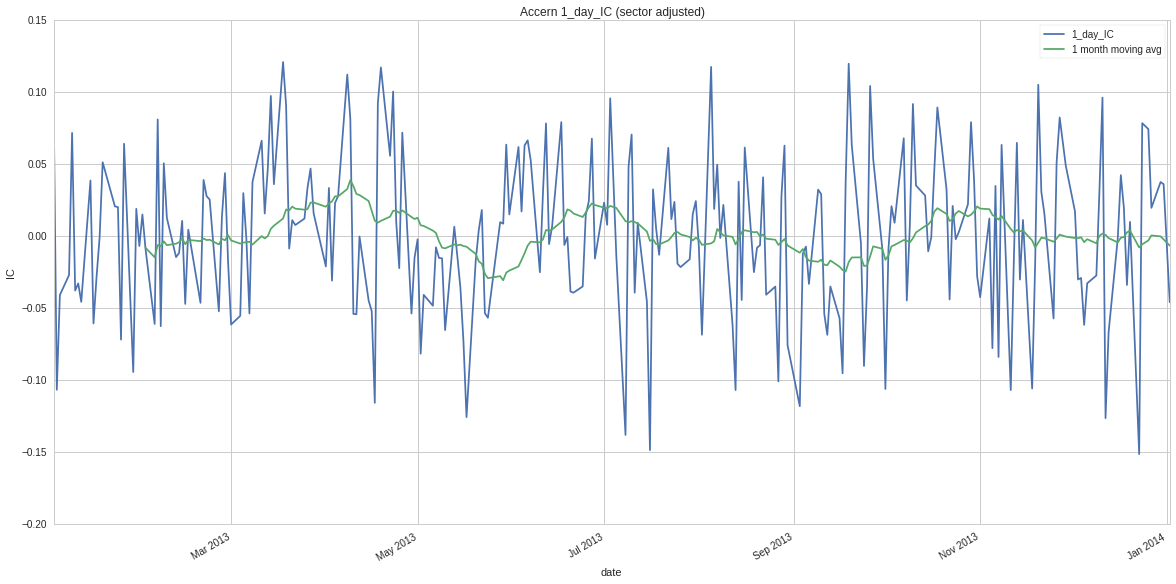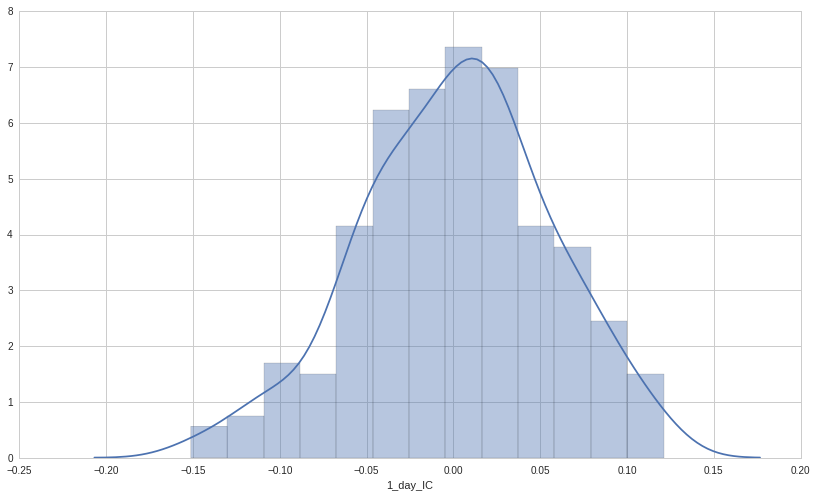5_day_IC mean: -0.00483025584013
5_day_IC stdev: 0.0488097728508
5_day_IC mean/stdev: -0.0989608342347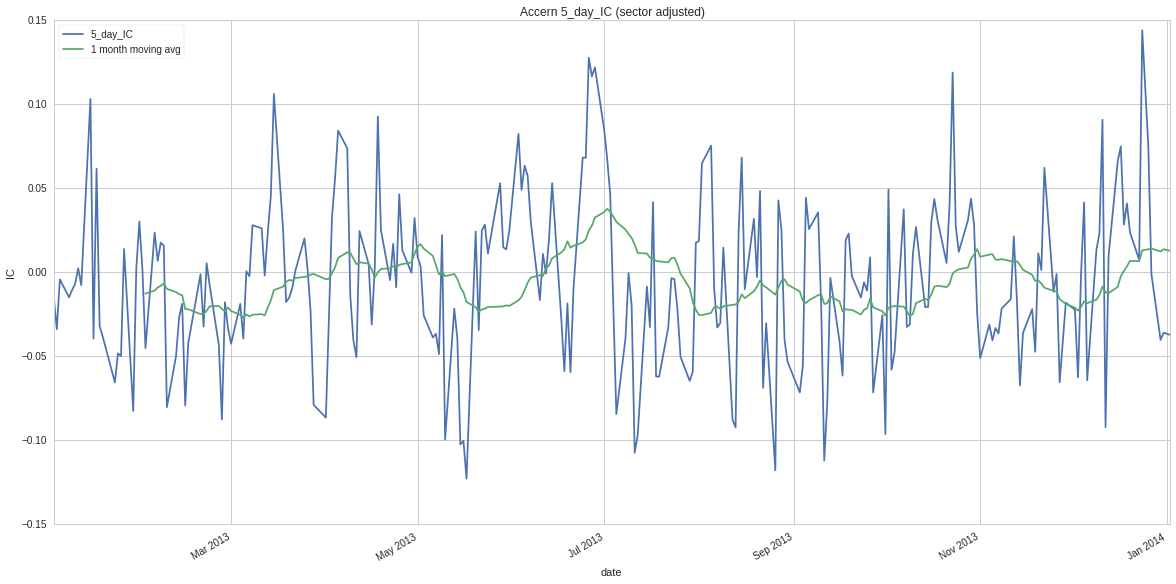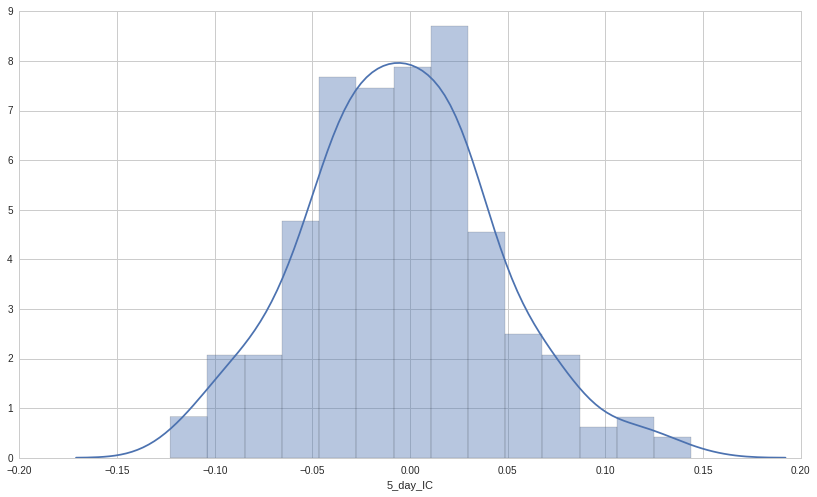20_day_IC mean: -0.0105962628317
20_day_IC stdev: 0.0482791770465
20_day_IC mean/stdev: -0.219478944753